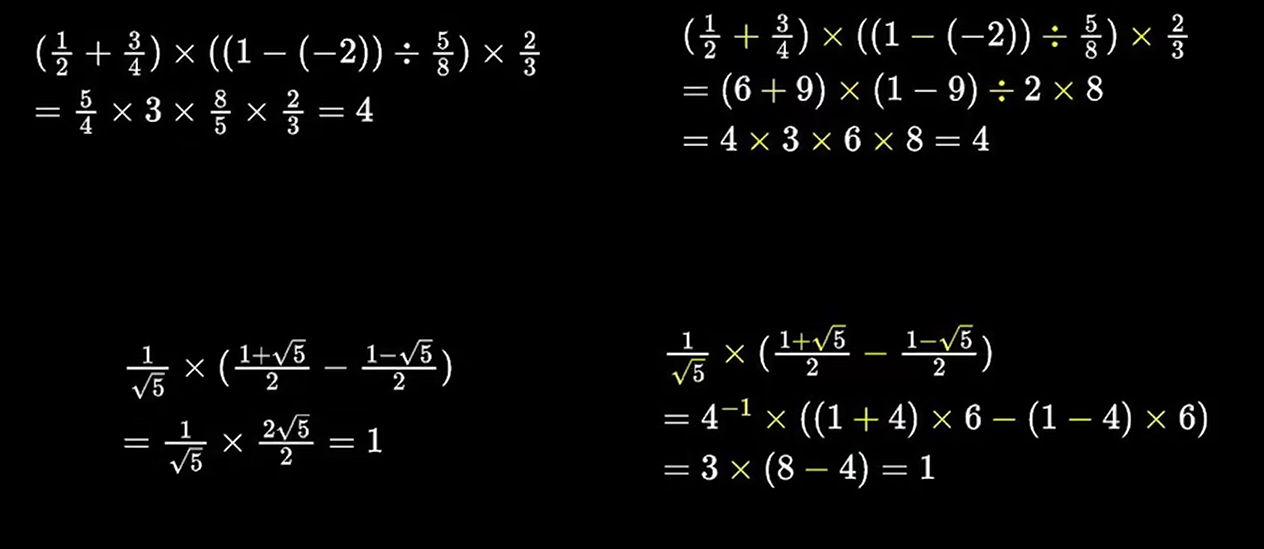# 如何在计算机底层将一部分浮点数运算，转换为等价的整数运算，从而大幅提高计算效率？

## 正文

​ 首先我需要默认读者有模运算基础, 如果没有, 请不要忽略这一部分

​ 首先我们都知道普通的实数域下的加法如何计算, 这里就不搞一遍加法的精确定义了; 然后减法是加相反数, 实数域下求相反数比较简单, 只需要加一个负号, 我们后面会讲如何定义一个相反数运算

1. 我们需要找到一个足够大的质数p, 已知我们求解的问题的结果一定小于这个足够大的质数, 在一般情形下,大于1024的质数就可以胜任很多环境的工作了
2. 我们运算的结果已知是整数, 但是运算过程中会参杂入浮点数, 比如计算斐波那契数列, 通项公式中参杂大量分数运算, 但是结果一定为整数
3. 计算式子中可以包含根号, 但是根号下的内容只能有一种, 因为在我们新定义的乘法中,

\sqrt{a}\sqrt{b}\neq\sqrt{ab}

a\oplus b = a+b\mod 11
a\otimes b = a\times b\mod 11

-a = 11-a \mod 11

6+7=13
\
6\oplus7=2
\
6\times7=42
\
6\otimes7=9
\
-6=-6
\
\ominus6=5
\
3^{-1}=\frac{1}{3}
\
3^{\ominus1}=4
\
7\div10=\frac{7}{10}
\
7\div10= 7\otimes10^{\ominus1}=7\times10=4(这个是精髓)## 参考资料

https://www.bilibili.com/video/BV1EM41117Dv